Name:    2nd Grade Math Basic Test 7

Multiple Choice
Identify the choice that best completes the statement or answers the question.

1.

Statistics/Probability and Graphing – Probability and Prediction – RIT 161 -170
On which number is the spinner most likely to land?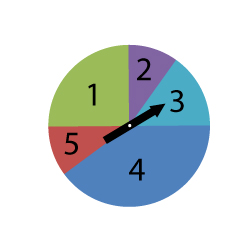a. 1 d. 4 b. 2 e. 5 c. 3

2.a. Lane d. Al b. Kay e. Kim c. Sue

3.

Statistics/Probability and Graphing – Graphing – RIT 171 – 180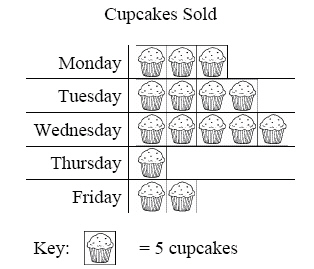How many cupcakes were sold on Wednesday?
 a. 52 d. 25 b. 15 e. 5 c. 3

4.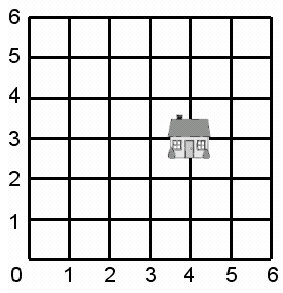The house is at what point on the grid?
 a. (4,4) d. (3,2) b. (2,2) e. (3,4) c. (4,3)

5.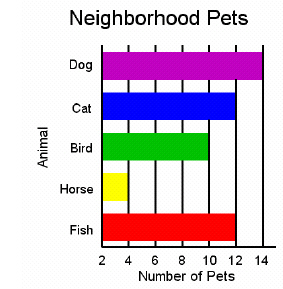Which pet did the most children have?
 a. Dog d. Horse b. Cat e. Fish c. Bird

6.

Statistics/Probability and Graphing – Probability and Prediction – RIT 171 –180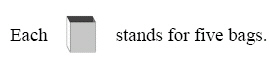How many bags do you think will be collected on Friday?
 a. 14 d. 20 b. 30 e. 25 c. 6

7.

Joan is making a quilt. She puts 4 stars in the first row, 5 stars in the second
row, and 4 stars in the third row.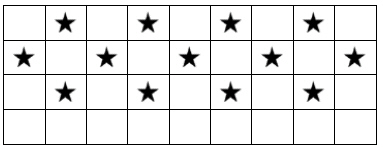How many stars will Joan put in the fifth row?
 a. 4 d. 3 b. 5 e. 13 c. 6